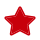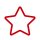# 复杂数据类型

Spark SQL支持复杂数据类型，如表1所示。

ARRAY

array(<value>,<value>[, ...])

MAP

map(K <key1>, V <value1>, K <key2>, V <value2>[, ...])

STRUCT

struct(<value1>,<value2>[, ...])

#### 使用限制

• 创建含有复杂数据类型字段的表时，该表存储格式不支持CSV（txt）。
• 如果表中含有复杂数据类型字段时，该表不支持CSV（txt）格式的文件数据导入。
• MAP数据类型建表必须指定schema，且不支持date、short、timestamp数据类型。
• 对于JSON格式OBS表，MAP的键类型只支持STRING类型。
• 由于MAP类型的键不能为NULL，MAP键不支持对插入数据进行可能出现NULL值类型之间的隐式转换，如：STRING类型转换为其他原生类型、FLOAT类型转换为TIMESTAMP类型、其他原生类型转换为DECIMAL类型等。
• STRUCT数据类型不支持double，boolean数据类型。

#### ARRAY示例

1. 创建表。

CREATE TABLE array_test(name STRING, id ARRAY < INT >) USING PARQUET;

2. 插入测试数据。

INSERT INTO array_test VALUES ('test',array(1,2,3,4));

INSERT INTO array_test VALUES ('test2',array(4,5,6,7))

INSERT INTO array_test VALUES ('test3',array(7,8,9,0));

3. 查询结果。

“array_test”表中的所有数据：

SELECT * FROM array_test;

```test3	[7,8,9,0]
test2	[4,5,6,7]
test	[1,2,3,4]```

“array_test”表中id数组第0个元素的数据。

SELECT id FROM array_test;

```7
4
1```

#### MAP示例

1. 创建表。

CREATE TABLE map_test(id STRING, score map<STRING,INT>) USING PARQUET;

2. 插入测试数据。

INSERT INTO map_test VALUES ('test4',map('math',70,'chemistry',84));

INSERT INTO map_test VALUES ('test5',map('math',85,'chemistry',97));

INSERT INTO map_test VALUES ('test6',map('math',88,'chemistry',80));

3. 查询结果。

查询“map_test”表里的所有数据。

SELECT * FROM map_test;

```test6	{"chemistry":80,"math":88}
test5	{"chemistry":97,"math":85}
test4	{"chemistry":84,"math":70}```

查询“map_test”表中的数学成绩。

SELECT id, score['Math'] FROM map_test;

```test6	88
test5	85
test4	70```

#### STRUCT示例

1. 创建表。

CREATE TABLE struct_test(id INT, info STRUCT<name:STRING,age:INT>) USING PARQUET;

2. 插入测试数据。

INSERT INTO struct_test VALUES (8, struct('zhang',23));

INSERT INTO struct_test VALUES (9, struct('li',25));

INSERT INTO struct_test VALUES (10, struct('wang',26));

3. 查询结果。

查询“struct_test”表中的所有数据。

SELECT * FROM struct_test;

```8	{"name":"zhang","age":23}
10	{"name":"wang","age":26}
9	{"name":"li","age":25}```

查询“struct_test”表中的nameage数据。

SELECT id,info.name,info.age FROM struct_test;

```8	zhang	23
10	wang	26
9	li	25```

# 相关产品